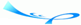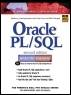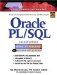# Chapter 1 Programming Concepts

 Team-FlyOracle® PL/SQL® Interactive Workbook, Second EditionBy Benjamin Rosenzweig, Elena Silvestrova Table of Contents Appendix D.  Answers to Test Your Thinking Sections

 1) Create the following structure: Based on the value of a number, determine if it is even or odd. Hint: Before you decide how to define even and odd numbers, you should decide what structure must be used to achieve the desired results. A1: Answer: Your answer should look similar to the following: ` IF MOD(NUMBER, 2) = 0 DISPLAY 'THIS NUMBER IS EVEN' IF MOD(NUMBER, 2) != 0 DISPLAY 'THIS NUMBER IS ODD' `In this example, you are using the selection structure because a decision whether a number is even or odd must be made. This decision can be made with the help of the built-in function MOD. This function returns the remainder of the NUMBER divided by 2. If a number is divisible by 2 (in other words, there is no remainder), then it is an even number. Otherwise, a number is an odd number.Assume that the number is equal to 16. The value returned by the MOD(16,2) is equal to 0. So the selection structure displays a message 'THIS NUMBER IS EVEN'. Next, assume that the number is equal to 7. The value returned by MOD(7,2) is equal to 1. So the select structure displays a message 'THIS NUMBER IS ODD'. 2) Create the following structure: The structure you created in the previous exercise is designed to work with a single number. Modify it so that it can work with a list of numbers. A2: Answer: Your answer should look similar to the following: ` WHILE THERE ARE MORE NUMBERS IF MOD(NUMBER, 2) = 0 DISPLAY 'THIS NUMBER IS EVEN' IF MOD(NUMBER, 2) != 0 DISPLAY 'THIS NUMBER IS ODD' GO TO THE NEXT NUMBER `This structure is a combination of two structures: iteration and selection. The iteration structure repeats its steps for each number in the list. The selection structure makes a decision based on a particular number.Assume that you have three numbers in your list: 10, 25, and 36. You start with the first number, 10. There are two more numbers left in the list. Next, the control of the flow is passed to the selection structure. Because the current number equals 10, the value returned by the MOD function is equal to 0. As a result, the message 'THIS NUMBER IS EVEN' is displayed. Then the control of the flow passed back to the iteration structure, and you are ready to move to the next number. The next number is equal to 25, and the value retuned by the MOD function is equal to 1. As a result, the message 'THIS NUMBER IS ODD' is displayed. Next, the control of the flow is passed back to the iteration structure to process the last number in the list, 36. This is an even number, so the selection structure displays the message 'THIS NUMBER IS EVEN'.

 Team-FlyTopOracle PL/SQL Interactive Workbook (2nd Edition)
ISBN: 0130473200
EAN: 2147483647
Year: 2002
Pages: 146

Similar book on Amazon

flylib.com © 2008-2017.
If you may any questions please contact us: flylib@qtcs.net